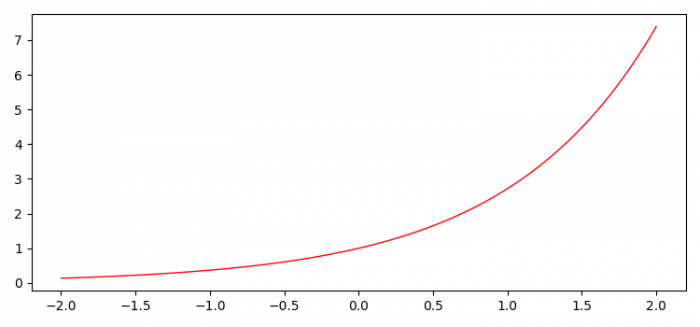# Adjusting the spacing between the edge of the plot and the X-axis in Matplotlib

To adjust the spacing between the edge of the plot and the X-axis, we can use tight_layout() method or set the bottom padding of the current figure.

• Set the figure size and adjust the padding between and around the subplots.
• Create x and y data points using numpy.
• Plot x and y data points using plot() method.
• To display the figure, use show() method.

## Example

import numpy as np
import matplotlib.pyplot as plt

plt.rcParams["figure.figsize"] = [7.50, 3.50]
plt.rcParams["figure.autolayout"] = True

x = np.linspace(-2, 2, 100)
y = np.exp(x)
plt.plot(x, y, c='red', lw=1)
plt.show()

## Output